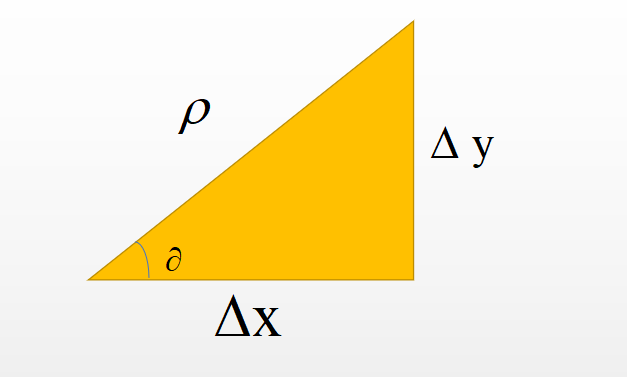• 本发明具体涉及一种基于梯度方向的图像边缘检测方法。背景技术：边缘检测是图像处理的基本问题，在图像分割、特征提取、视觉导航等领域有广泛的应用。基于微分的边缘检测算法如Sobel算子、Prewitt算子、Laplace算子...

本发明具体涉及一种基于梯度方向的图像边缘检测方法。

背景技术：

边缘检测是图像处理的基本问题，在图像分割、特征提取、视觉导航等领域有广泛的应用。基于微分的边缘检测算法如Sobel算子、Prewitt算子、Laplace算子和Canny算子等，因计算高效而被广泛应用。在Canny算子的基础上，研究者们做了一些有意义的改进。在Canny构架的启发下，很多研究者构造了一些新型卷积核用来检测边缘。然而微分算子对噪声、光照变化、对比度变化等因素极为敏感，虽然人们进行了一些改进，但仍没有较好地解决这个问题。有学者从频率域的角度出发，提出了相位一致性的概念。相位一致性不受灰度梯度的影响，所以能较好地抵抗光照变化与对比度变化。但是此类算法一方面计算量很大，很难应用于实时系统，另一方面对于大尺度模糊边缘不敏感，所以没有得到广泛的应用。

事实上空间域也存在一致性现象，即局部区域内的梯度方向一致性。有学者最早将局部边缘一致性的概念应用于边缘检测算法的评估，但该方法依赖于阈值筛选以后的结果，所以无法独立完成边缘检测任务。

技术实现要素：

本发明要解决的技术问题是提供一种基于梯度方向的图像边缘检测方法。

基于梯度方向的图像边缘检测方法，包括以下步骤：

S1：设置用于计算合成梯度方向和ISGD的阈值T，计算8个方向的梯度方向和，并确定每个像素点的；

S2：计算Canny梯度，并进行非极大值抑制，将非极大值抑制确定的点集作为初始边缘点集；

S3：用Canny梯度确定弱边缘候选区；

S4：确定基础阈值；

S5：计算与ISGD相关的权重；

S6：计算与ISGD的规律性相关的权重；

S7：基础阈值乘以权重，进行阈值的自适应调节，并确定最终的边缘图。

进一步的，ISGD的计算方法如下：

1)图像区域内的灰度，其水平方向梯度值，梯度计算方法如下式：

2)对灰度梯度进行量化，给定点(i,j)处水平方向梯度方向计算公式为：

其中，T为确定是否存在梯度方向的判定阈值；

3)设计领域灰度梯度方向和，称为梯度方向和(SGD)指标，水平方向(i,j)处SGD值定义为：

4)设计合成梯度方向和(ISGD)指标，水平方向和竖直方向的ISGD计算方法如下：

其中，分别为右上、右下、左下方向的梯度方向和。

进一步的，的计算方法如下：

为8个方向的ISGD最大值，是描述梯度方向一致性的梯度方向和指标。

进一步的，所述弱边缘候选区的确定方法如下：

以像素(i,j)为中心，观察窗口长度为L范围内所有像素点的梯度幅值，如果水平或竖直方向上连续有n个点的梯度幅值小于梯度阈值，则判定该点属于弱边缘候选区；n取值为5，设为10。

进一步的，基础阈值的确定方法如下：

1)中的基础阈值取低基础阈值，非中取高基础阈值，即,

;

2) 低基础阈值取值为/2,即为5；

3)高基础阈值计算方法如下：

3-1)首先，以(i,j)为中心的的邻域内，对方向一致性好的点进行统计，并求出它们的梯度均值，计算公式如下：

其中，为的邻域内大于6的点的集合，表示集合中元素个数，为梯度幅值；

3-2)其次，将标识弱边缘的阈值作为最小门限，选取搞基础阈值为：

进一步的，计算与ISGD相关的权重如下：

式中，m是高斯分布的均值也是均匀分布的取值，σ为高斯分布的标准差。

进一步的，计算与ISGD的规律性相关的权重如下：

将统计区域内ISGD大于12的像素点数量与大于6的像素点数量之比叫做ISGD的降速比，用RI表示，则，

本发明的有益效果是：

本发明利用真实图像边缘两侧的灰度渐变性，以及边缘点周围灰度梯度的方向一致性好而非边缘点周围灰度梯度的方向一致性差的特点构造了梯度方向和(SGD)指标，并根据该指标提出一种阈值自适应的边缘检测算法。梯度方向和在有效提取边缘点的同时能较好地抑制高强噪声，该指标对光照和对比度变化有较强的鲁棒性，该方法能较好地解决兼顾弱边缘检测的同时而不引入噪声干扰的问题。

具体实施方式

以下具体实施例对本发明作进一步阐述，但不作为对本发明的限定。

基于梯度方向的图像边缘检测方法，包括以下步骤：

S1：设置用于计算合成梯度方向和ISGD的阈值T，计算8个方向的梯度方向和，并确定每个像素点的；

S2：计算Canny梯度，并进行非极大值抑制，将非极大值抑制确定的点集作为初始边缘点集；

S3：用Canny梯度确定弱边缘候选区；

S4：确定基础阈值；

S5：计算与ISGD相关的权重；

S6：计算与ISGD的规律性相关的权重；

S7：基础阈值乘以权重，进行阈值的自适应调节，并确定最终的边缘图。

ISGD的计算方法如下：

1)图像区域内的灰度，其水平方向梯度值，梯度计算方法如下式：

2)对灰度梯度进行量化，给定点(i,j)处水平方向梯度方向计算公式为：

其中，T为确定是否存在梯度方向的判定阈值；

3)设计领域灰度梯度方向和，称为梯度方向和(SGD)指标，水平方向(i,j)处SGD值定义为：

4)设计合成梯度方向和(ISGD)指标，水平方向和竖直方向的ISGD计算方法如下：

其中，分别为右上、右下、左下方向的梯度方向和。

的计算方法如下：

为8个方向的ISGD最大值，是描述梯度方向一致性的梯度方向和指标。

所述弱边缘候选区的确定方法如下：

以像素(i,j)为中心，观察窗口长度为L范围内所有像素点的梯度幅值，如果水平或竖直方向上连续有n个点的梯度幅值小于梯度阈值，则判定该点属于弱边缘候选区；n取值为5，设为10。

基础阈值的确定方法如下：

1)中的基础阈值取低基础阈值，非中取高基础阈值，即,

;

2) 低基础阈值取值为/2,即为5；

3)高基础阈值计算方法如下：

3-1)首先，以(i,j)为中心的的邻域内，对方向一致性好的点进行统计，并求出它们的梯度均值，计算公式如下：

其中，为的邻域内大于6的点的集合，表示集合中元素个数，为梯度幅值；

3-2)其次，将标识弱边缘的阈值作为最小门限，选取搞基础阈值为：

计算与ISGD相关的权重如下：

式中，m是高斯分布的均值也是均匀分布的取值，σ为高斯分布的标准差。

计算与ISGD的规律性相关的权重如下：

将统计区域内ISGD大于12的像素点数量与大于6的像素点数量之比叫做ISGD的降速比，用RI表示，则，

展开全文• 就比如我们学梯度下降时，都知道梯度下降是机器学习、深度学习的核心优化算法，但为什么沿梯度方向，函数变化最快呢？？？ 问了不少人都不能说的很清楚，所以查阅了很多博客，视频，现分享给大家。 导数 导数的几何...

很多时候，我们时间有限，对一些知识只能不求甚解，但这这些不求甚解的知识又会很困扰我们，总想着原理是啥，为啥这样做。就比如我们学梯度下降时，都知道梯度下降是机器学习、深度学习的核心优化算法，但为什么沿梯度方向，函数变化最快呢？？？

问了不少人都不能说的很清楚，所以查阅了很多博客，视频，现分享给大家。

导数

导数的几何意义可能很多人都比较熟悉: 当函数定义域和取值都在实数域中的时候，导数可以表示函数曲线上的切线斜率。 除了切线的斜率，导数还表示函数在该点的变化率。

比如中学学到的加速度 a = Δ v Δ t a=\frac{\Delta v}{\Delta t} ，这表示的是速度v对时间t的导数，物理意义表示的是速度变化的快慢。

放到数学上也是一样， 除了切线的斜率，导数还表示函数在该点的变化率也就是变化快慢。在一元函数中，只有一个自变量变动，也就是说只存在一个方向的变化率，也就没有沿哪个方向变化快慢的问题了。下面延伸到多元函数，以二元函数为例。

偏导数

沿x方向的方向导数：
l i m Δ x → 0 f ( x 0 + Δ x , y 0 ) − f ( x 0 , y 0 ) Δ x = ∂ z ∂ x (1) lim_{\Delta x\rightarrow0}\frac{f(x_0+\Delta x,y_0)-f(x_0,y_0)}{\Delta x}=\frac{\partial z}{\partial x}\tag{1}
移动下位置：
l i m Δ x → 0 f ( x 0 + Δ x , y 0 ) − f ( x 0 , y 0 ) = ∂ z ∂ x Δ x (2) lim_{\Delta x\rightarrow0}{f(x_0+\Delta x,y_0)-f(x_0,y_0)} =\frac{\partial z}{\partial x}{\Delta x}\tag{2}

沿y方向的方向导数：
l i m Δ y → 0 f ( x 0 , y 0 + Δ y ) − f ( x 0 , y 0 ) Δ y = ∂ z ∂ y (3) lim_{\Delta y\rightarrow0}\frac{f(x_0,y_0+\Delta y)-f(x_0,y_0)}{\Delta y}=\frac{\partial z}{\partial y}\tag{3}
移动下位置：
l i m Δ y → 0 f ( x 0 , y 0 + Δ y ) − f ( x 0 , y 0 ) = ∂ z ∂ y Δ y (4) lim_{\Delta y\rightarrow0}{f(x_0,y_0+\Delta y)-f(x_0,y_0)} =\frac{\partial z}{\partial y}{\Delta y} \tag{4}

2+4:
l i m Δ y → 0 l i m Δ x → 0 f ( x 0 + Δ x , y 0 + Δ y ) − f ( x 0 , y 0 ) = ∂ z ∂ y Δ y + ∂ z ∂ x Δ x (5) lim_{\Delta y\rightarrow0}lim_{\Delta x\rightarrow0}{f(x_0+\Delta x,y_0+\Delta y)-f(x_0,y_0)} =\frac{\partial z}{\partial y}{\Delta y} +\frac{\partial z}{\partial x}{\Delta x}\tag{5}同时除 ρ \rho

l i m Δ y → 0 l i m Δ x → 0 f ( x 0 + Δ x , y 0 + Δ y ) − f ( x 0 , y 0 ) ρ = ∂ z ∂ y Δ x ρ + ∂ z ∂ x Δ y ρ (6) lim_{\Delta y\rightarrow0}lim_{\Delta x\rightarrow0}\frac{{f(x_0+\Delta x,y_0+\Delta y)-f(x_0,y_0)}}{\rho} =\frac{\partial z}{\partial y}\frac{\Delta x}{\rho} +\frac{\partial z}{\partial x}\frac{\Delta y}{\rho}\tag{6}

l i m Δ y → 0 l i m Δ x → 0 f ( x 0 + Δ x , y 0 + Δ y ) − f ( x 0 , y 0 ) ρ = ∂ z ∂ y cos ⁡ α + ∂ z ∂ x sin ⁡ α (7) lim_{\Delta y\rightarrow0}lim_{\Delta x\rightarrow0}\frac{{f(x_0+\Delta x,y_0+\Delta y)-f(x_0,y_0)}}{\rho} =\frac{\partial z}{\partial y}\cos{\alpha} +\frac{\partial z}{\partial x}\sin{\alpha}\tag{7}

其中G为梯度，也就是沿x,y方向的导数组成的向量：
G = ( ∂ z ∂ y , ∂ z ∂ x ) G=(\frac{\partial z}{\partial y} ,\frac{\partial z}{\partial x})
I为方向导数的方向，这是一个单位向量
I = ( cos ⁡ α , sin ⁡ α ) I=(\cos{\alpha} ,\sin{\alpha})

方向导数：
方 向 导 数 = G ⋅ I = ∂ z ∂ y cos ⁡ α + ∂ z ∂ x sin ⁡ α = ∣ ∂ z ∂ y ∣ ∣ ∂ z ∂ x ∣ cos ⁡ θ 方向导数=G\cdot{I} =\frac{\partial z}{\partial y}\cos{\alpha} +\frac{\partial z}{\partial x}\sin{\alpha}= |\frac{\partial z}{\partial y} ||\frac{\partial z}{\partial x}| \cos{\theta}
θ \theta 为方向夹角
方向导数越大，表明函数上升越快，要想方向导数最大，即 cos ⁡ θ \cos{\theta} 要最大， cos ⁡ θ ∈ ( − 1 , 1 ) \cos{\theta}\in {(-1,1)} 也就是 θ = 0 \theta=0 时，方向导数最大，上升最快； θ = π \theta=\pi 时，方向导数为反方向最大，下降最快。

ok，以上证明完毕。

展开全文人工智能 机器学习 神经网络 逻辑回归 深度学习
• 我有一个160x120 OpenCV Mat，其中我在每个位置都存储了一个Vec3F向量。...在OpenCV中计算3D梯度方向然后我试图计算该对象中每个像素的渐变。我因此具有用C语言实现这个代码++/OpenCV的：for(int x ...

我有一个160x120 OpenCV Mat，其中我在每个位置都存储了一个Vec3F向量。 Vec3f保存用于已经通过使用强度图像和对应的深度图的3D重建计算的特定像素的信息。 所以我基本上从Mat映射到在每个像素保持3D位置信息的每个像素的灰度值到Mat。在OpenCV中计算3D梯度方向

然后我试图计算该对象中每个像素的渐变。我因此具有用C语言实现这个代码++/OpenCV的：

for(int x = 0; x < mat.rows; ++x){

for(int y = 0; y < mat.cols; ++y){

float Gx = (mat.at(x+1, y) - mat.at(x-1, y))/2.0;

float Gy = (depth.at(x, y+1) - depth.at(x, y-1))/2.0;

Vec3f d = (Gx, Gy, 1.0);

Vec3f n = normalize(d);

}

}

// mat.at(x, y) -> Get the Vec3F vector at the current x-, y-

// position in the Mat and access its first element (which is the points x-value).

因此，我所计算的梯度方向和Gx与Gy有限差分近似的方法。

我不明白的是如何计算Z方向的梯度。我确实有存储在Vec3f中的每个像素的z信息以及x和y信息，但是有限差分近似的步骤将不可能，因为数据存储在2D Mat中，对吧？

所以我不能简单地访问像素的前面和后面，他当前做类似这样的：在Mat

float Gz = (mat.at(x, y, z+1) - mat.at(x, y, z-1))/2.0;

，因为我还没有z值，对不对？如果是这样，那我该如何计算z方向上的梯度？我需要将我的信息存储在3D array中吗？或者整个方法不正确？谢谢！

+0

不确定您的2D渐变是否有意义。我知道3D体积数据(如CT扫描或密度三维重建(如kinect融合))等密度网格中的3D渐变。但是，如果需要，可以将稀疏点表示转换为3D网格。可能回答这个问题，你应该告诉你想要用梯度信息做什么。也许足够逼近该物体/像素位置中的二维表面平面。 –

展开全文• 梯度是机器学习里面的重要基础，借助梯度下降才能最小化损失函数，再逐步更新网络参数，得到最佳的函数表示。 梯度的概念是基于方向导数的， 单位向量的表示

梯度(本质上是一个向量）是机器学习里面的重要基础，借助梯度下降才能最小化损失函数，逐步更新网络参数，得到最佳的函数表示。梯度方向的变化率最大，沿着梯度的反方向，可以最大效率的降低损失函数。在对梯度的理解上，首先明确：先有方向导数的概念，才有梯度的定义

单位向量

以三维空间下为例，单位向量表示为：

分别是该单位向量与各坐标轴的夹角，通过3个夹角的约束，可以使该向量指向任何方向。且规定是单位向量，其模长为1

角度是表示该单位向量的最重要的部分，直接用表示单位向量感觉更直接，用cos表示是为了计算的方便？

方向导数及梯度

以二元函数为例，在点处，求解偏导数时候：

表示 f 在 x 方向的变化率

表示 f 在 y 方向的变化率。

但在该点下，可以朝各个方向运动，各个方向都有其各自的变化率，即方向导数。方向导数不能直接求导解出，可通过计算极限来求。

在任意方向上变化的长度，都要对应到各坐标轴上变化了多少，才能找到变化后的点坐标。

规定单位向量，在此方向上运动 t 个长度，则对应在x,y轴上的运动的长度分别为，在z方向产生的增量为

沿着方向的变化率为。（等式左边是极限表示下的变化率，通过方向导数的定理得出右边式子）

随着值的改变，函数可沿着任意方向运动，其变化率是，等价于两个向量做内积。

,,则有

其中是两个向量的夹角，可以看出当为1时候，变化率最大，即两向量平行时，也就是沿着（函数对其个变量偏导组成的向量）方向，变化率最大，将命名为梯度。

https://zhuanlan.zhihu.com/p/38525412

https://zhuanlan.zhihu.com/p/24913912

通过拉格朗日证明方向导数定理：https://zhuanlan.zhihu.com/p/66996168

展开全文• img_sobel_64x = cv2.Sobel(img_src, cv2.CV_64F, 1, 0) # 图像深度 64位浮点数 x方向一阶求导 img_sobel_64y = cv2.Sobel(img_src, cv2.CV_64F, 0, 1) # Gx Gy img_sobel_x = cv2.convertScaleAbs(img_sobel_64x...python opencv
• 要注意，导数是具有方向的。 一元函数的导数值和方向： ...至于梯度梯度是什么？我们规定曲面上该点下降幅度最大的反方向（还是下降幅度最大的那个方向所在的直线上，只不过是下降的反方向，也就...
• 梯度方向垂直于函数等值线的方向小唠嗑证明：梯度方向垂直于函数等值线的方向结尾小独白 小唠嗑 上一周整个人状态不太对劲，可能是碰到了很多杂七杂八的事情，人在矛盾当中就会陷入更深的矛盾，一直想一直想不到...线性代数 算法 深度学习 机器学习
• 方向导数与梯度 1 方向导数 定义 1： 设函数z=f(x,y)z=f(x,y)z=f(x,y)在点P(x,y)P(x,y)P(x,y)的某一领域U(P)U(P)U(P)内有定义，lll为自点PPP出发的射线，P′(x+Δx,y+Δy)P^{\prime}(x+\Delta x, y+\Delta y)P′(x+...
• 为什么梯度方向就是最速下降？  原理：泰勒展开  即：  -▽f(x)Δx 即df(x)Δx ，为函数值的变化量，是向量df(x) 和 Δx的点积，当两向量共线时点积值最大，因此要想找到从A点最快到B点的路径，就要找到最大点积...机器学习
• 同样的， 因此，无约束优化的问题可以简化为一个标准的二次规划（二次规划凸优化有个好处，就是，局部最优解即为全局最优解）， 其中， 下面是关于可行梯度方向法的核心观点: 可行梯度方向法的思想如下图所示。...算法
• 导数、方向导数与梯度

千次阅读 2021-01-17 18:29:03
导数，方向导数，切线、梯度是从高中就开始接触的概念，然而对这几个概念的认识不清，困惑了我很长时间，下面我将以图文并茂的形式，对这几个概念做详细的解释。1， 导数定义：设函数y=f(x)在点x0的某个邻域内有定义...
•负梯度 解释
• 没想到今天自己也要参与进来成为它的从业者，还有一点小兴奋哈哈，但是不得不说，它确实很吃数学底子，即使我考研数学将近130我也并没有很容易的就理解了它,今天就被一个问题给难住了，为什么梯度方向一定是沿着函数...机器学习 深度学习 人工智能
• 方向导数和梯度是什么？

千次阅读 2021-01-17 18:29:05
为什么梯度方向是函数在该点的方向导数最大的方向梯度的模是最大方向导数的值？大家在看复习全书时，有认真想过这个问题吗？小编在本文以二元函数为例详细讲解方向导数和梯度，并试图以尽可能通俗地语言回答上述...
• 在机器学习的过程中，求导是一件重要的基础数学工具。尤其是对于多特征的数据、模型而言...机器学习优化参数的一个重要方法就是在方向向量的基础上求其梯度。本文介绍了梯度方向导数的含义以及其在机器学习中的应用。机器学习 人工智能 深度学习 神经网络 随机梯度下降
• 二、方向梯度直方图流程2.1 预先归一化（Normalization）2.2 计算梯度2.2.1 水平方向和垂直方向上的梯度2.2.2 计算梯度的幅值和方向2.3 方向梯度直方图2.3.1 平均分割2.3.2 构建方向梯度直方图2.4 区域（Blocks）...人工智能 深度学习 机器学习 计算机视觉 目标跟踪
• 梯度下降法是数学优化中比较常用的方法，在机器学习中也有不少应用。 函数 F(x1,x2,…,xn)F(x_1, x_2, \dots, x_n)F(x1​,x2​,…,xn​) 的梯度就是它的一阶导数： ΔF=(∂F∂x1,∂F∂x2,…,∂F∂xn) \Delta F=(\...梯度下降 法线 等高线 垂直
• 如果不愿意看文字描述的，这里推荐一个视频 方向导数和梯度的直观理解。 什么是梯度？？ 可以这么想象一下，大地平面为XOY所在平面(z=0)，你现在处于山坡上的某点P(x,y,z),你怎样才能最快时间跑下山坡呢? 分析一下：...数学
• 方向导数和梯度概念辨析

千次阅读 2021-03-29 23:42:29
方向导数和梯度概念辨析 我们在学习多元函数的时候，经常会困惑于方向导数和梯度的区别以及他们的几何意义，今天让我们来一起辨析一下。 一、什么是方向导数？ 定义：函数的增量 f(x+△x,y+△y)−f(x,y)f(x+\...
• HOG(Histogram of Oriented Gradient)方向梯度直方图，主要用来提取图像特征，最常用的是结合svm进行行人检测。算法流程图如下(这篇论文上的)： 下面我再结合自己的程序，表述一遍吧：1.对原图像gamma校正，img=sqrt...
• 上面的例子很容易理解，但接下来不一样： 此处的梯度方向角为165度，模长为85，如何映射至不同分箱？ 首先确定模长的比例：由于165位于160到180之间，其中160-165段占25%，165-180段占75%，因此模长85可以分解为 85*...
• 导数，反映的是函数 y=f(x)y=f(x)y=f(x) 在某一点处沿xxx轴正方向的变化率。公式定义如下： 再强调一遍，导数f’(x)f’(x)f’(x)是函数f(x)f(x)f(x)在xxx轴上某一点处沿着xxx轴正方向的变化率。 直观地看，也就是在...人工智能 机器学习 偏导数 方向导数 梯度
• 方向导数与梯度

千次阅读 2020-12-18 20:53:56
沿不同方向的变化率就是方向导数问题，而下降的最快方向就是梯度问题。 顾名思义，方向导数就是某个方向上的导数。 什么是方向？ 我们知道： 函数f(x,y)的A点在这个方向上也是有切线的，其切线的斜率就是方向方向导数 梯度
• 方向梯度直方图(HOG)中，梯度方向分布被用作特征。沿着一张图片X和Y轴的方向上的梯度是很有用的，因为在边缘和角点的梯度值是很大的，我们知道边缘和角点包含了很多物体的形状信息。 怎么计算方向梯度直方图呢？ ...
• 方向导数是数，而梯度是向量。 梯度是函数相对一个向量包含所有偏导数的向量，记为 ∇xf(x)。... 以某点为中心，有无数个方向导数，但是只有一个最大，就是沿着梯度方向的那个。 方向导数是梯度在这个方向上的投影。深度学习 神经网络
• 刚接触梯度下降这个概念的时候，是在学习机器学习算法的时候，很多训练算法用的就是梯度下降，然后资料和老师们也说朝着梯度的反方向变动，函数值下降最快，但是究其原因的时候，很多人都表达不清楚。所以我整理出...
• 三、方向导数与梯度的关系。四、从泰勒展开式的角度理解。五、从方向导数的角度理解。偏导的几何意义：平行于x轴或y轴方向的垂直平面上截线的斜率。方向导数的几何意义：就是某一方向上垂直平面截线在该方向的斜率 ...
• 1.梯度是导数的高维形式，导数是增长的方向，所以梯度其实是增长的方向，那么，梯度的反方向就是增长的反方向，下降 2.梯度方向导数取得最大值的方向
• 基于梯度方向、极化变换和聚类算法的图像主特征直线检测 代码主要思路： 1）借助类LSD直线检测，提取图像各个方向梯度； 2）对像素中的各个梯度方向做极化变换； 3）对计划变换后的结果进行聚类运算； 4）对...
• 方向导数，然后才能了解梯度和为什么梯度方向是函数值上升最快的方向 对于导数，我们要明确，导数除了代表函数在某点的切线的斜率外，还表示函数在该点的变化率 f(x0)′=limΔx→0ΔyΔx=limΔx→0f(x0+Δx)−f(x0)...机器学习...